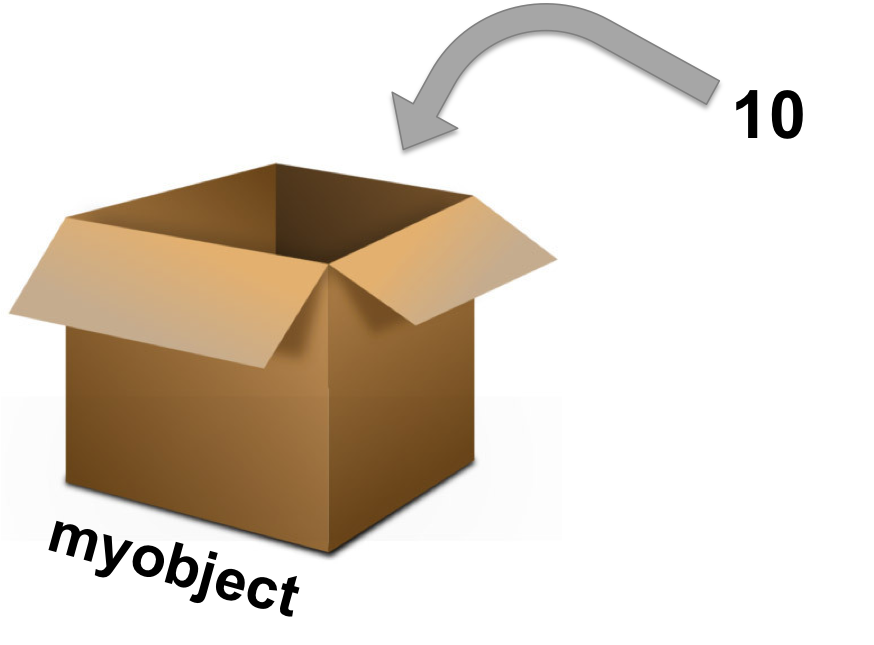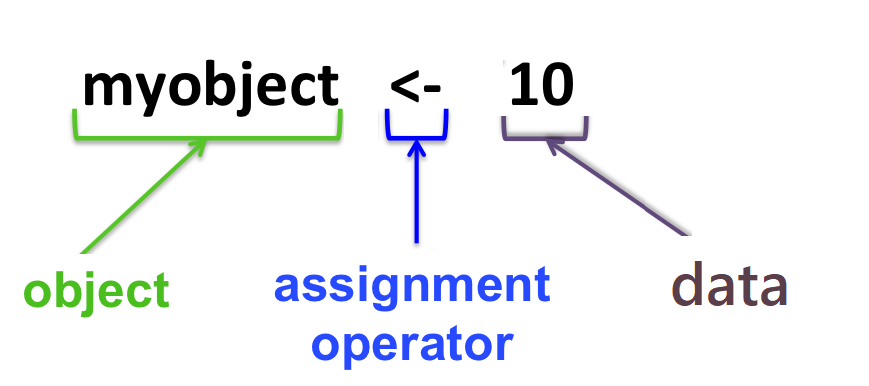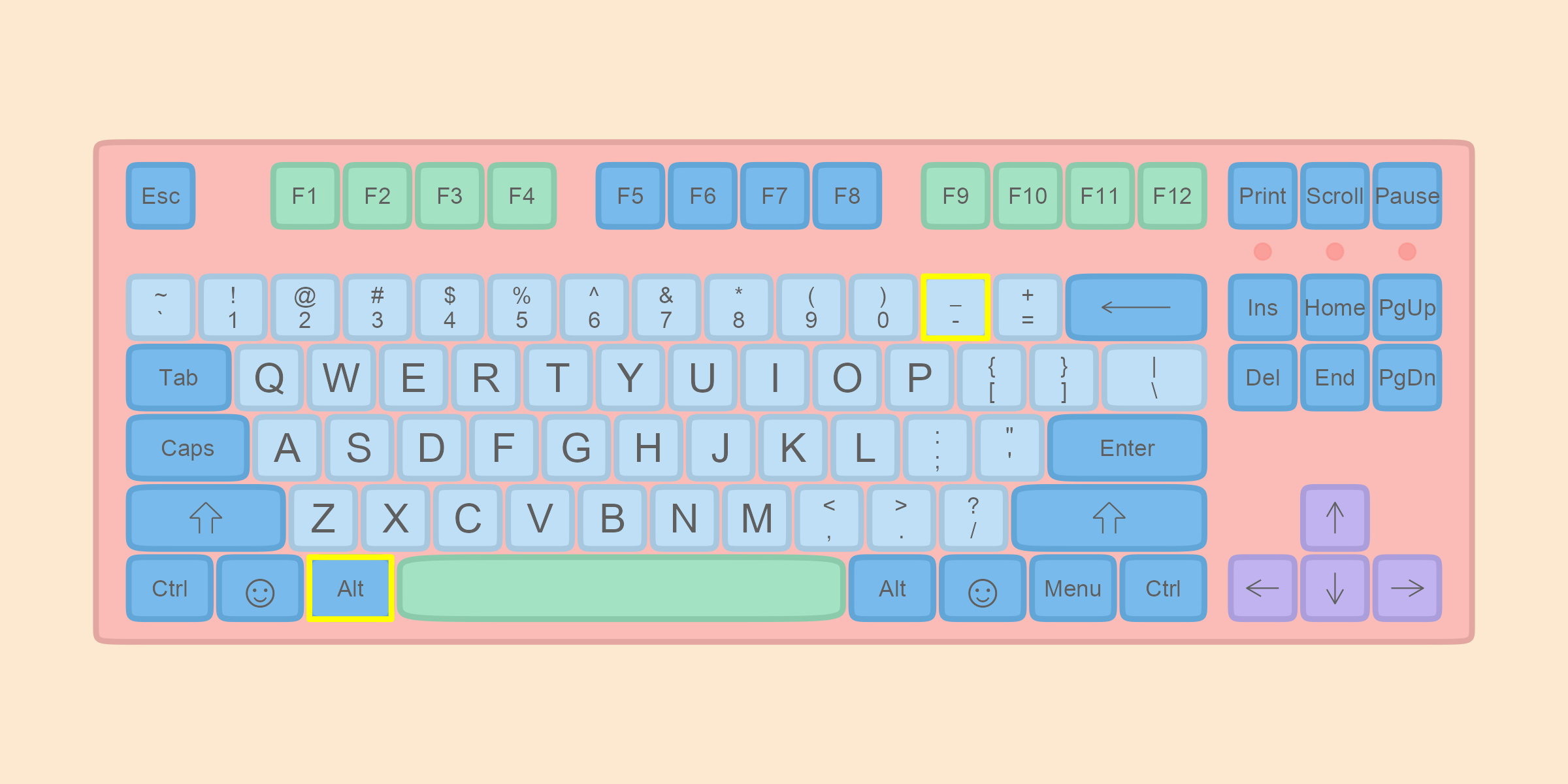# 第 3 章 对象

## 3.1 基本运算

1 + 2
5 - 3
3 * 4
12 / 3
2^3
(2 + 4) / 3

## 3.2 对象

### 3.2.1 一切都是对象### 3.2.2 对象的创建与使用x <- 5

• alt-（windows系统）
• option-（苹果系统）x
##  5

x + 2
##  7

x
##  5

x <- 6

x
##  6

### 3.2.3 变量命名规则

R变量名必须以字母、数字、下划线_和句点.组成。但是，变量名的第一个字符不能为数字或者特殊符号，比如 +, -, *, /, ^, !, @, &, 或者下划线_。变量名的第一个字符如果是句点.，那么句点后面不能紧跟数字。变量名是区分大小写的，yY是两个不同的变量名。在中文环境下，汉字也可以作为变量名的合法字符使用，但不推荐使用。

• 大小写敏感
• 不能有空格，可以用下划线代替空格，比如，my_age <- 30
• 开头不能是数字和一些特殊符号

• 一般的变量名
x <- 175
• 不错变量名
height <- 175
• 更佳的变量名
boy_height_cm <- 175

### 3.2.4 对象属性

typeof(x) 
##  "double"
length(x)
##  1

## 3.3 习题

• example <- c(1, 2, 3)
• example1 <- c(1, 2, 3)
• example.1 <- c(1, 2, 3)
• example_1 <- c(1, 2, 3)
• example-1 <- c(1, 2, 3)
• example+1 <- c(1, 2, 3)
• .example <- c(1, 2, 3)
• .2example <- c(1, 2, 3)
• 2example <- c(1, 2, 3)
• _example <- c(1, 2, 3)